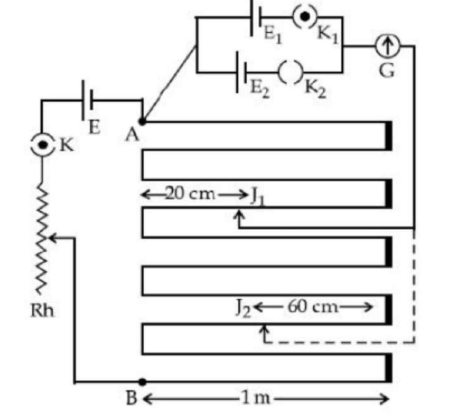# In the given circuit of potentiometer,Question:

In the given circuit of potentiometer, the potentital difference $\mathrm{E}$ across $A B(10 \mathrm{~m}$ length $)$ is larger

than $\mathrm{E}_{1}$ and $\mathrm{E}_{2}$ as well. For key $\mathrm{K}_{1}$ (closed), the jockey is adjusted to touch the wire at point $J_{1}$ so that there is no deflection in the galvanometer. Now the first battery $\left(E_{1}\right)$ is replaced by second battery $\left(E_{2}\right)$ for working by making $K_{1}$ open and $E_{2}$ closed. The galvanometer gives then null deflection at $\mathbf{J}_{2}$. The value of $\frac{\mathrm{E}_{1}}{\mathrm{E}_{2}}$ is $\frac{\mathrm{a}}{\mathrm{b}}$, where $\mathrm{a}=$Solution:

(1)

$\frac{E_{1}}{E_{2}}=\frac{I_{1}}{l_{2}}$\

$=\frac{3 \times 100 \mathrm{~cm}+(100-20) \mathrm{cm}}{7 \times 100 \mathrm{~cm}+60 \mathrm{~cm}}$

$=\frac{380}{760}=\frac{1}{2}=\frac{a}{b}$

$a=1$